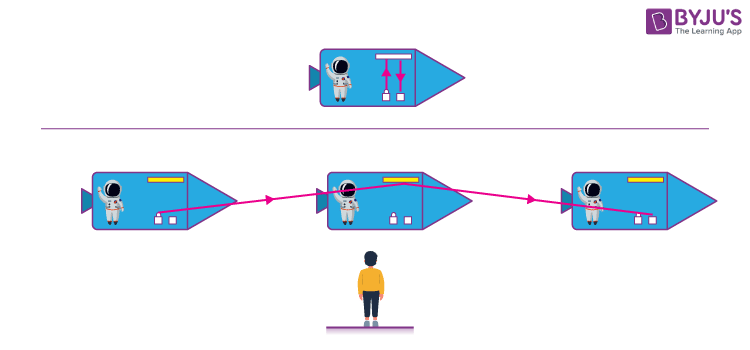# Relativity

## Introduction to Relativity

Relativity is a theorem formulated by Albert Einstein, which states that space and time are relative, and all motion must be relative to a frame of reference. It is a notion that states’ laws of physics are the same everywhere. This theory is simple but hard to understand.

It states:

• There is no absolute reference frame. One can measure velocity if the object or momentum is only in relation to other objects.
• The speed of light is constant irrespective of who measures it or how fast the person measuring it is moving.

Albert Einstein’s Theory of Relativity encompasses two theories: Special Relativity Theory and General Relativity Theory.

## Special Theory of Relativity

Einstein first introduced this term in the year 1905. It is a theorem that deals with the structure of space-time. Einstein explained this theory based on two postulates –

• The laws of physics are the same for all, irrespective of the observer’s velocity.
• The speed of light is always constant regardless of the motion of the light source or the motion of the observer.This is the theory which laid the foundation of time travel. According to Einstein, the rate at which time tics decreases with the increase of the person’s velocity. But this is hard to notice as the decrease in time is relatively very low compared to the increase in time. So, it can be assumed that if you can equal the velocity of light, you will be in a situation where time is still. This phenomenon is called Time Dilation. There are other surprising consequences of this theory, such as –

• Relativity of simultaneity – two actions, simultaneous for one person, may not be simultaneous for another person in relative motion.
• Length Shrinking: Objects are measured and appear shorter in the direction they are moving with respect to the observer.
• Mass – Energy Equivalence: Study of relativity led to one of the greatest inventions, i.e., E = mc2 where E is Energy, m stands for mass and c for the velocity of light. Many scientists observed that the object’s mass increases with the velocity but never knew how to calculate it. This equation is the answer to their problem, which explains that the increased relativistic weight of the object is equal to the kinetic energy divided by the square of the speed of light.

## General Theory of Relativity

General Relativity theory, developed by Einstein in 1907-1915, states that being at rest in the gravitational field and accelerating are identical physically. For example, an observer can see the ball fall the same way on the rocket and on Earth. This is due to the rocket’s acceleration, which equals 9.8 m/s2. This theory relates to Newton’s gravitational theory and special relativity.### Some Consequences of General Relativity are :

• Gravitational Time Dilation: Gravity influences the passage of time. Clocks in the deeper gravitational wells run slower than in general gravitational levels.
• Light rays will bend in the gravitational field.
• The universe is expanding, and parts of it are moving away from Earth faster than the speed of light.

Stay tuned with BYJU’S to know more about the theory of relativity, its applications and other interesting topics like relative speed, relative velocity, relative motion, etc.

## However large or small, everything falls because of gravity. But somehow, the moon seems to be unaffected. Have you wondered why?